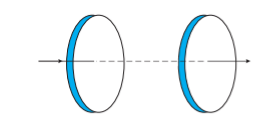Home/Class 12/Physics/

## QuestionPhysicsClass 12

Figure shows a capacitor made of two circular plates each of radius  $$12\mathit{cm}$$ and separated by  $$5.0\mathit{cm.}$$  The capacitor is being charged by an external source (not shown in the figure). The charging current is constant and equal to  $$0.15A$$ . Obtain the displacement current across the plates.0.15 A
4.64.6## Solution

Distance between the capacitor plates,  $$d=5\mathit{cm}=0.05m$$
Radius of circular plate,  $$r=12\mathit{cm}=0.12m$$
Constant charging current,  $$I=0.15A$$
Displacement current =  $$0.15A$$
As displacement current is equal to conduction current across the capacitor plates.